ISSN: 1070-4698

# Vol. 39

Latest Volume
All Volumes
All Issues

PIER Vol. 39, 299-339, 2003. doi:10.2528/PIER02112501

###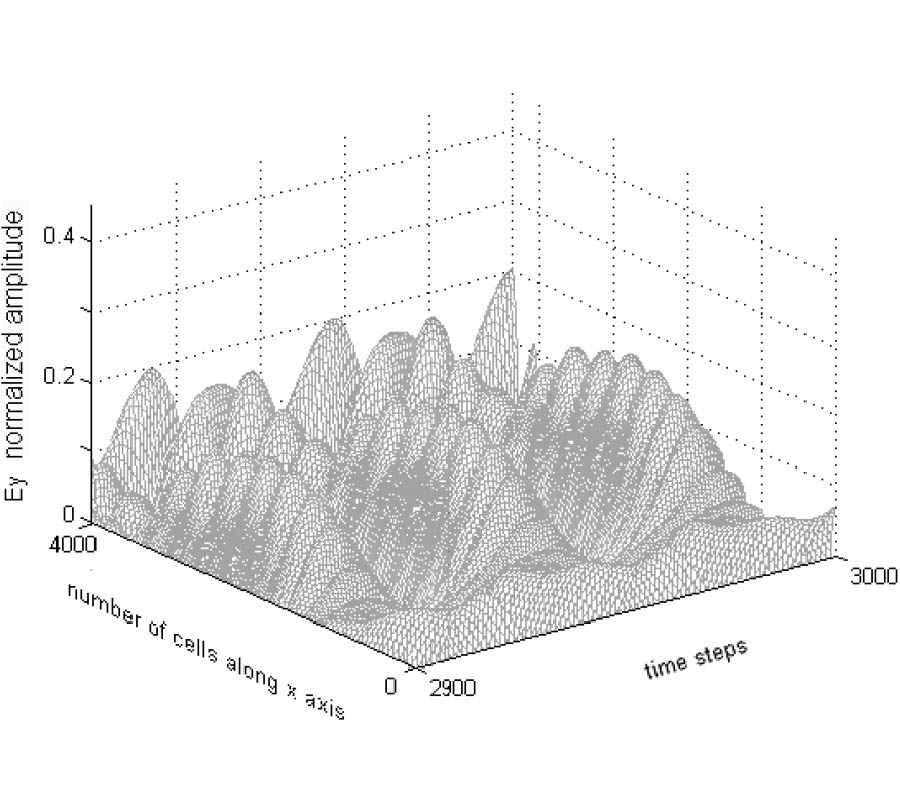Finite Difference Time Domain Modeling of Light Amplification in Active Photonic Band Gap Structures

Abstract-The paper deals with the modeling, based on the Finite Difference Time Domain method, of active one- and twodimensional photonic crystals. The onset of laser oscillation is observed by simulating the active substance as having a negative frequency-dependent Lorentzian-shaped conductivity so including into Maxwell's equations an electric current density. Particular attention is devoted to the implementation of uniaxial perfectly matched layer absorbing boundary conditions for the simulation of infinitely extending structures having gain features. Laser behaviour is simulated as a function of various parameters; the threshold wavelengthand conductivity are evaluated as the wavelengthand conductivity where the transmittance diverges. Moreover, the properties of the active two-dimensional photonic band gap structures are given in terms of a Q quality factor which increases by increasing the crystal size and strongly depends on the lattice shape. For the square lattice, when the crystal size increases from N = 2 to N = 8 the Q-factor increases by about an order of magnitude (from 0.027 to 0.110) for TE polarization while for TM polarization it decreases from 0.025 to 0.022. At last the Q-factor pertaining to the chess-board lattice, to parity of other parameters, assumes greater values and already for N = 4, it reaches the values obtained for the 16×8 square lattice, for bothTE and TM polarizations.

PIER Vol. 39, 281-298, 2003. doi:10.2528/PIER02112101

###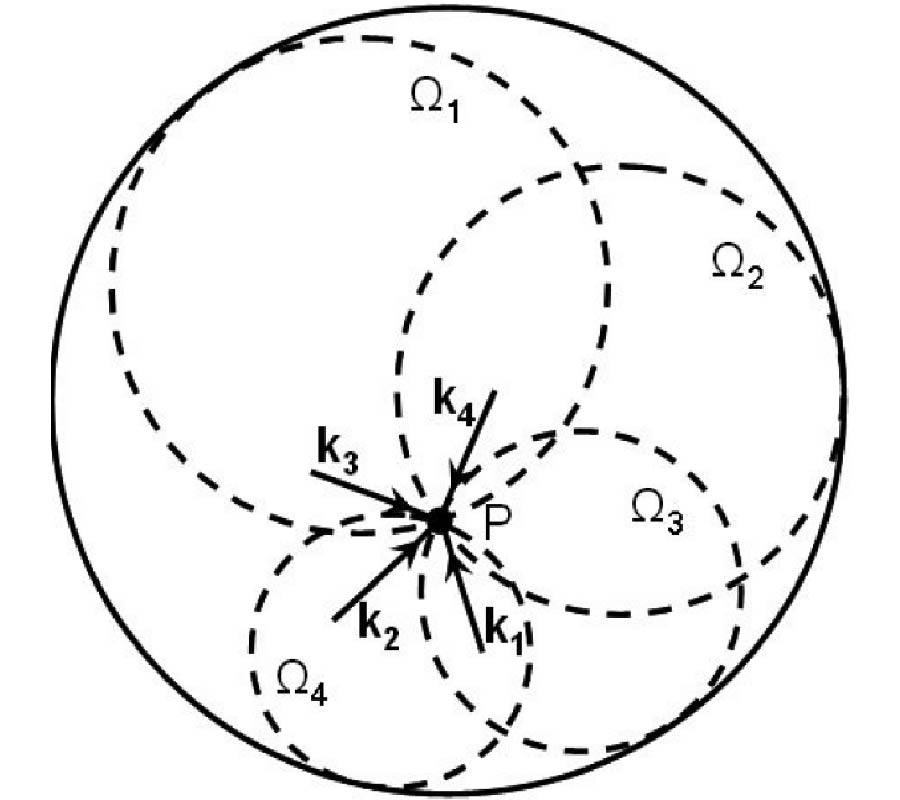Topological Wavelength Shifts [Electromagnetic Field in Lobachevskian Geometry]

It is shown that in hyperbolic spaces, an electromagnetic radiation experiences shifts in spectrum as a function of curvature and distance. The equation relating distance in hyperbolic space, its curvature, and spectral shift is derived by method of horospheres. The active nature of the Lobachevskian vacuum is discussed with applications to physics.

PIER Vol. 39, 265-279, 2003. doi:10.2528/PIER02111902

###Modes in a Hard Surface Waveguide with Uniaxially Anisotropic Chiral Material Filling

Propagation of waves in circular waveguide withth e boundary condition of hard surface is considered. The waveguide is filled with uniaxial chiral material. This study is a generalization of previously studied cases withisotropic chiral or anisotropic material filling. The eigenvalue equation is formed and the corresponding eigenmodes are presented. It is seen that the hard surface boundary condition simplifies the field analysis remarkable. While the eigenwaves in anisotropic waveguide were TE and TM fields in this more general case the eigenwaves are elliptically polarized hybrid fields. Since the eigenwaves are certain combinations of TE and TM fields and propagate withdifferen t propagation factors, uniaxial chiral medium can be used for polarization transformation. Reflection and transmission from a uniaxial chiral section of a waveguide is analyzed withn umerical examples.

PIER Vol. 39, 249-264, 2003. doi:10.2528/PIER02093001

###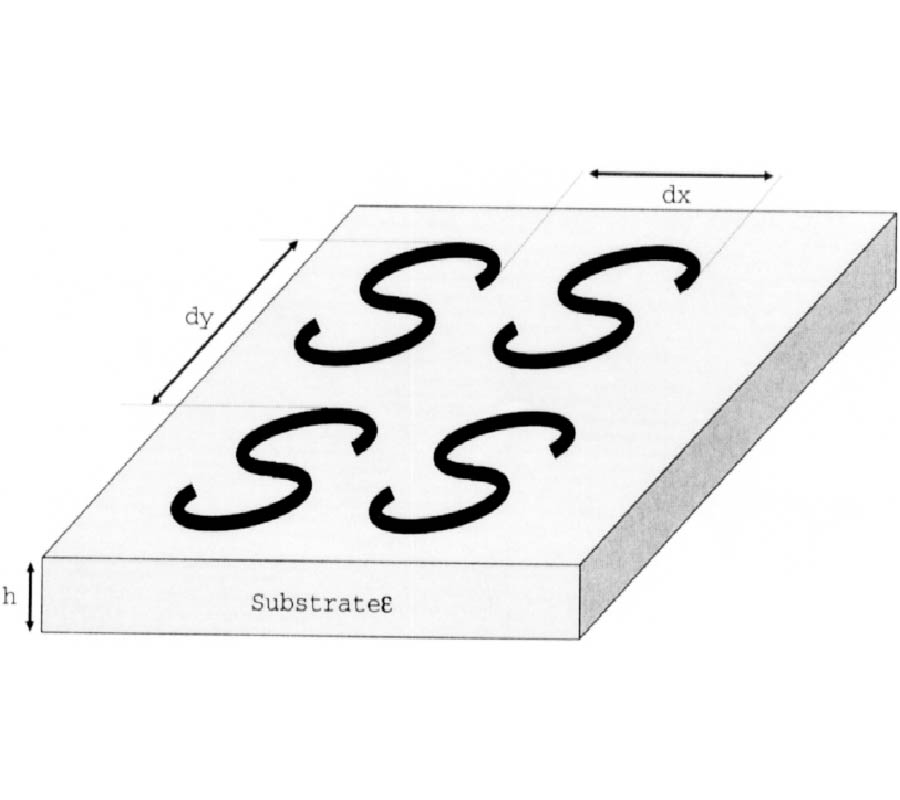Homogenization of an Array of S-Shaped Particles Located on a Dielectric Interface

An analytical model of a grid composed of small S-shaped conducting particles located on the surface of a dielectric slab is presented. This approach replaces the original one-layer structure with metallic particles printed on the interface by a multilayered structure with homogenized permittivities for each layers. This way one can homogenize the arrays of small resonant particles. The analytical model is verified by numerical simulations for the case of normal incidence of the plane wave. The homogenization is possible due to the small sizes of S-particles compared to the resonant wavelength in the substrate and due to the small thickness of the whole structure.

PIER Vol. 39, 193-247, 2003. doi:10.2528/PIER02070605

###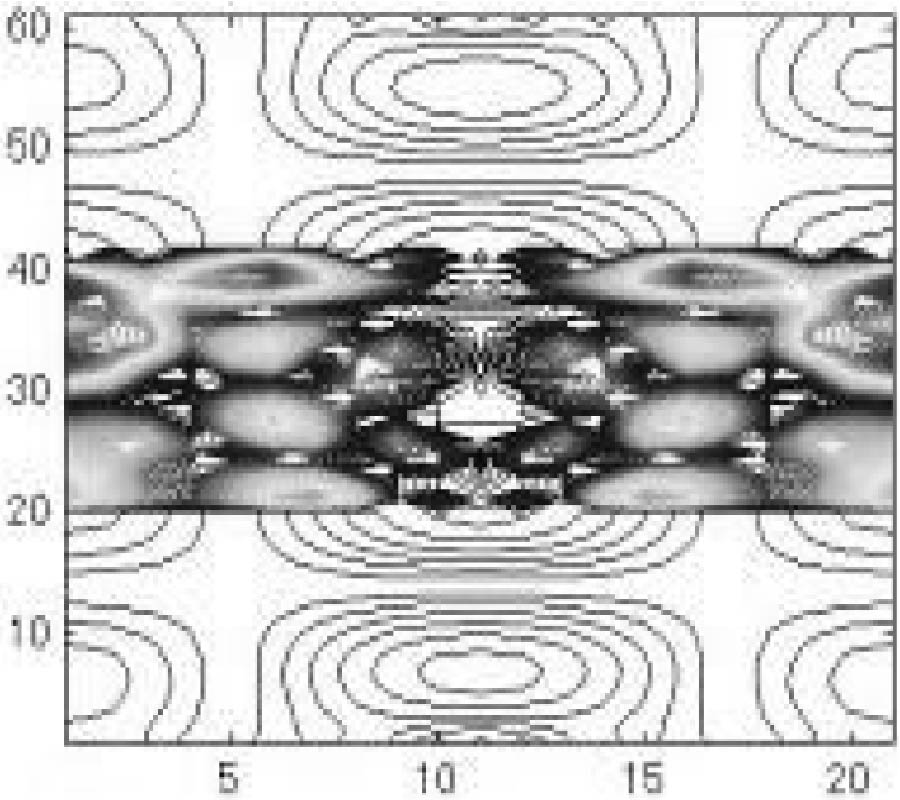Oscillations in Slotted Resonators with Several Slots: Application of Approximate Semi-Inversion

We consider oscillations in cylindrical slotted resonators formed by combinations of rectangular domains with several slots cut in the walls using the methods of approximate semi-inversion of integral operator-valued functions with a logarithmic singularity of the kernel. The initial boundary value problems for the Helmholtz equation are reduced to Fredholm integral equations and systems of integral equations of the first kind with a logarithmic singularity. In the case of narrow slots, the dispersion equations are obtained and evaluated using perturbations and the small-parameter method. Eigenfrequencies and eigenfields are calculated explicitly. The values of geometrical and material parameters are determined that lead to the interaction of oscillations. The results obtained are used for improving the design of filters and switches on the basis of simple model prototype structures.

PIER Vol. 39, 177-192, 2003. doi:10.2528/PIER02062602

###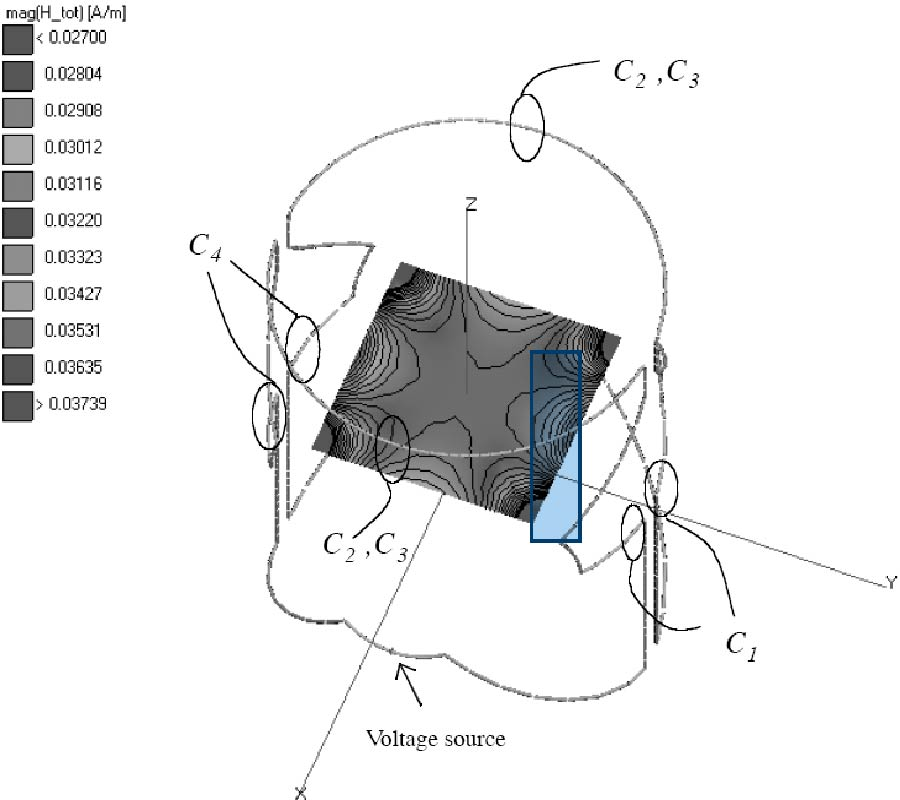A Genetic Algorithm/Method of Moments Approach to the Optimization of an RF Coil for MRI Applications --- Theoretical Considerations

A Combined Genetic Algorithm and Method of Moments design methods is presented for the design of unusual near-field antennas for use in Magnetic Resonance Imaging systems. The method is successfully applied to the design of an asymmetric coil structure for use at 190 MHz and demonstrates excellent radiofrequency field homogeneity.

PIER Vol. 39, 147-175, 2003. doi:10.2528/PIER02060902

###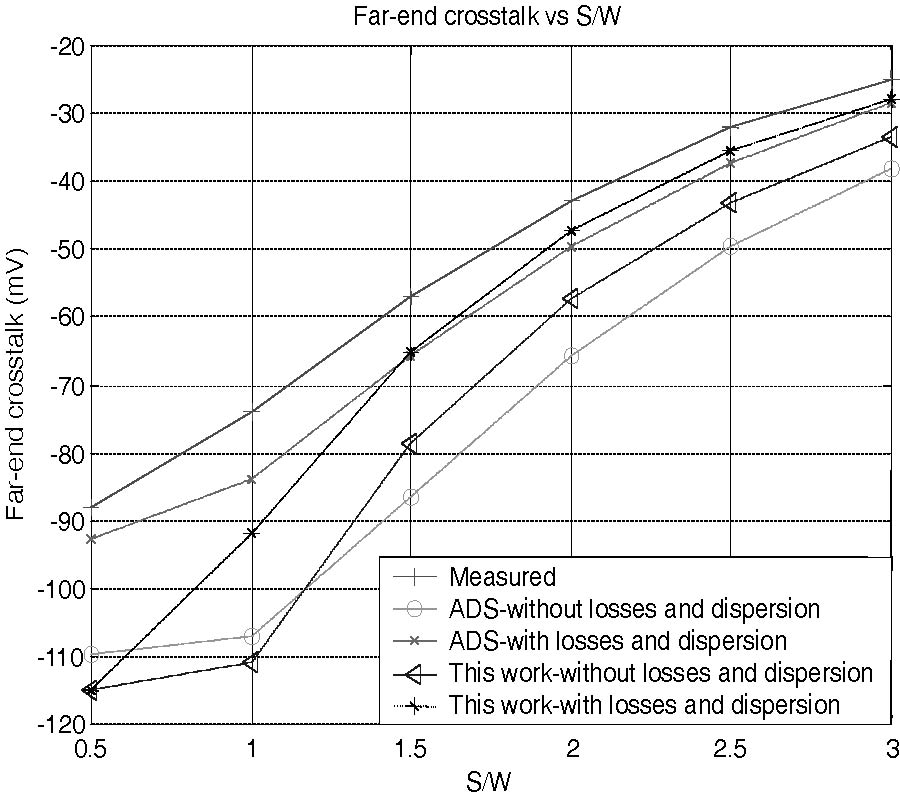Simple Time-Domain Expressions for Prediction of Crosstalk on Coupled Microstrip Lines

This paper presents an improved variant of timedomain method for predicting crosstalk on parallel-coupled matched terminated microstrip lines. This method derives simple near-end and far-end time-domain crosstalk expressions which are applicable to lossless case with significant harmonic frequency < 1 GHz. The expressions are in polynomial form with geometrical dimensions of the structure and stimulus information as the only required entry parameters. They are simpler as compared to other methods because the difficult-to-determine distributed RLCG electrical parameters of the coupled lines are not needed. A look-up table for the polynomial coefficients is generated for easy application of this technique. The expressions are applicable for board thickness of 4-63 mils, 30-70Ω line characteristic impedance, 0.5W-4.0W (where W is the line width) inner edge to edge separation, and 3-5 dielectric constant. For significant harmonic frequency > 1 GHz, the effect of both losses and dispersion on the crosstalk levels is accounted for by investigating the gradient of the distorted driving signal. The peak crosstalk levels are then predicted by modifying the time derivative term in the lossless expressions. In addition, the far-end crosstalk is proved to saturate at half of the magnitude of the driving signal entering the active line. The saturation phenomenon is studied from the viewpoint of difference in odd-mode and even-mode propagation velocities.

PIER Vol. 39, 125-145, 2003. doi:10.2528/PIER02053002

###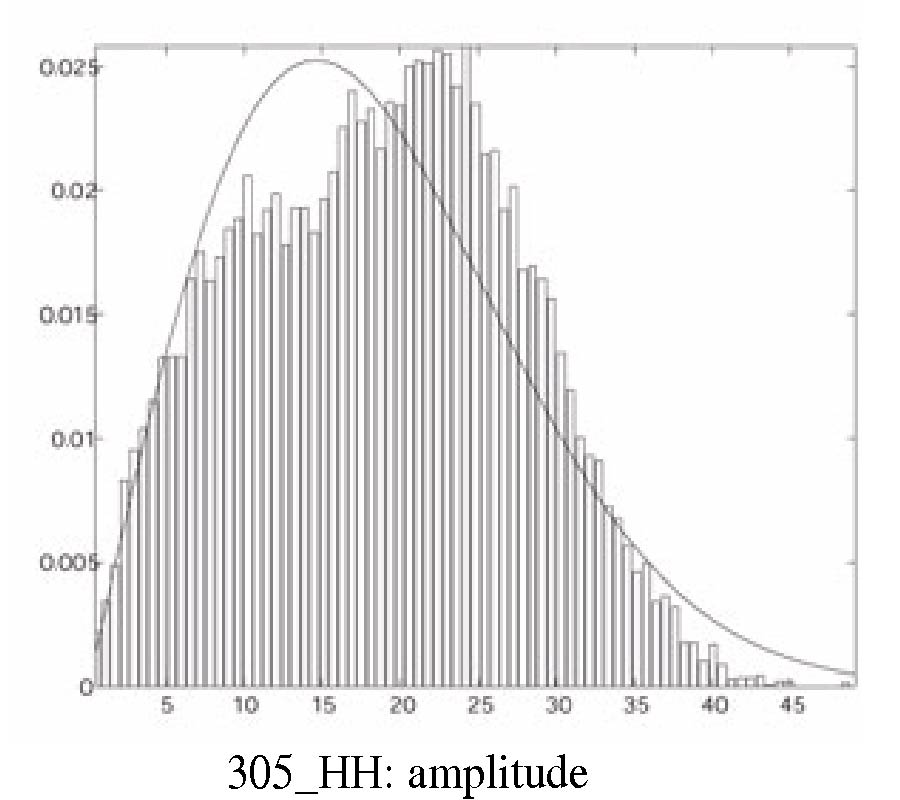Compression of Polarimetric Synthetic Aperture Radar Data

The paper deals with proposition and evaluation of new and specific methods to represent vector radar data acquired by means a side-looking measurement in order to use compression process of Lind, Buzo, Gray (LBG), and Kohonen's self organizing feature maps of topology. The aim is to enable after coding, transmission, and decoding a high-resolution reconstruction image using the Synthetic Aperture Radar (SAR) methods. The approach proposed for compression uses the statistical properties of the signals to be compressed in order to perform the vector quantification in an optimal way.

PIER Vol. 39, 93-123, 2003. doi:10.2528/PIER02052408

###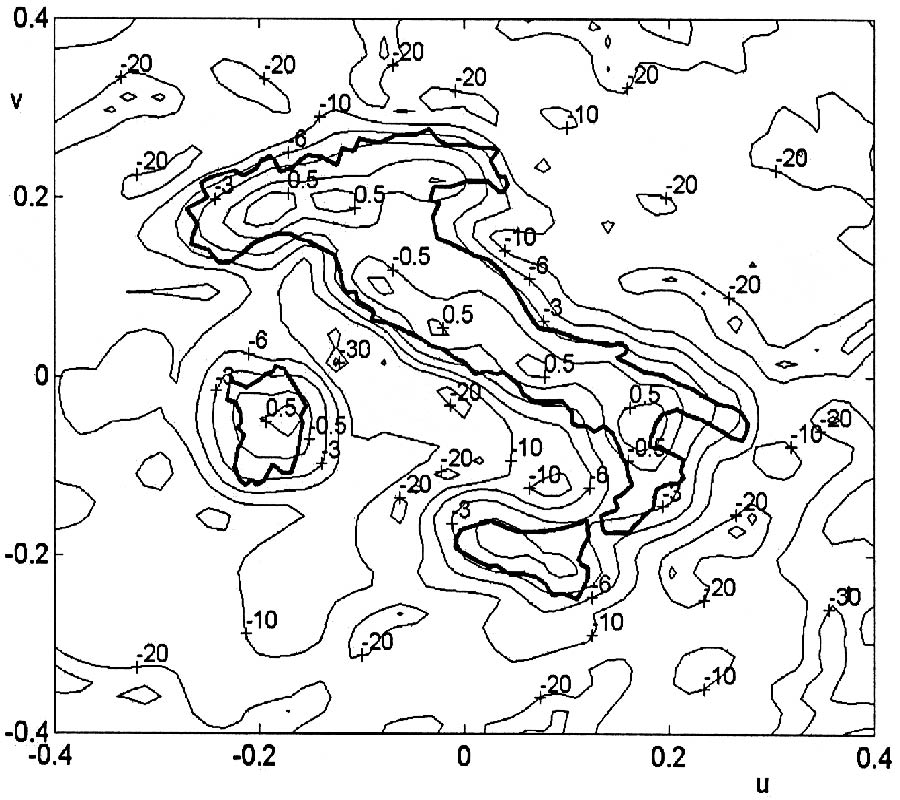An Effective Power Synthesis Technique for Shaped, Double-Reflector Multifeed Antennas

A new synthesis algorithm for shaped, double-reflector antennas with complex array feed is presented. The approach presented here aims to improve the efficiency of synthesis techniques without missing the required accuracy. The algorithm is based on a convenient splitting of the original problem into two phases, each one involving a sub-problem significantly simpler than the original one. A double reflector synthesis problem involving only Fourier Transform (FT) operators is of concern during the first phase. The subre- flector surface and a first estimate of the main reflector geometry are obtained in this step. A single reflector synthesis problem is considered during the second phase wherein the final main reflector surface and the excitation coefficients of the primary feed array are obtained. While in the first phase only approximate relationships between the unknowns and the secondary radiated field are exploited, in the second phase accurate radiation operators are involved. Despite this accuracy, the second phase is still numerically effective since it involves a single reflector synthesis problem and exploits, as "good" starting point, the main reflector estimate obtained during the first phase. The effectiveness of the approach is due to the fact that the necessity of dealing simultaneously with two reflector surfaces, the key of the synthesis difficulties, is afforded only during the first phase where efficient computational tools are allowed. A numerical example shows the effectiveness of the proposed approach.

PIER Vol. 39, 61-91, 2003. doi:10.2528/PIER02082205

###Green's Function Expansions in Dyadic Root Functions for Shielded Layered Waveguides

Dyadic Green's functions for inhomogeneous parallel-plate waveguides are considered. The usual residue series form of the Green's function is examined in the case of modal degeneracies, where secondorder poles are encountered. The corresponding second-order residue contributions are properly interpreted as representing "associated functions" of the structure by constructing a new dyadic root function representation of the Hertzian potential Green's dyadic. The dyadic root functions include both eigenfunctions (corresponding to first-order residues) and associated functions, analogous to the idea of Jordan chains in finite-dimensional spaces. Numerical results are presented for the case of a two-layer parallel-plate waveguide.

PIER Vol. 39, 47-59, 2003. doi:10.2528/PIER02050804

###FDTD Modeling of a Vibrating Intrinsic Reverberation Chamber

The field conditions inside a vibrating intrinsic reverberation chamber (VIRC) are examined. By the use of the Finite Difference Time Domain (FDTD) method,the field strength in the VIRC is calculated,and an investigation of the field uniformity and the field distribution is performed. The modes inside the cavity are excited by applying an appropriately modulated waveform on a dipoles gap. The use of this kind of waveform enables the study of the field conditions over a wide frequency range. On the contrary,an implementation of the field excitation with an unmodulated carrier would require a simulation of the FDTD method at each frequency of interest. Thus,a considerable reduction in the simulation time is achieved. The results presented,describing the field behavior inside the enclosure,agree with theory to a high degree.

PIER Vol. 39, 1-45, 2003. doi:10.2528/PIER02083001

###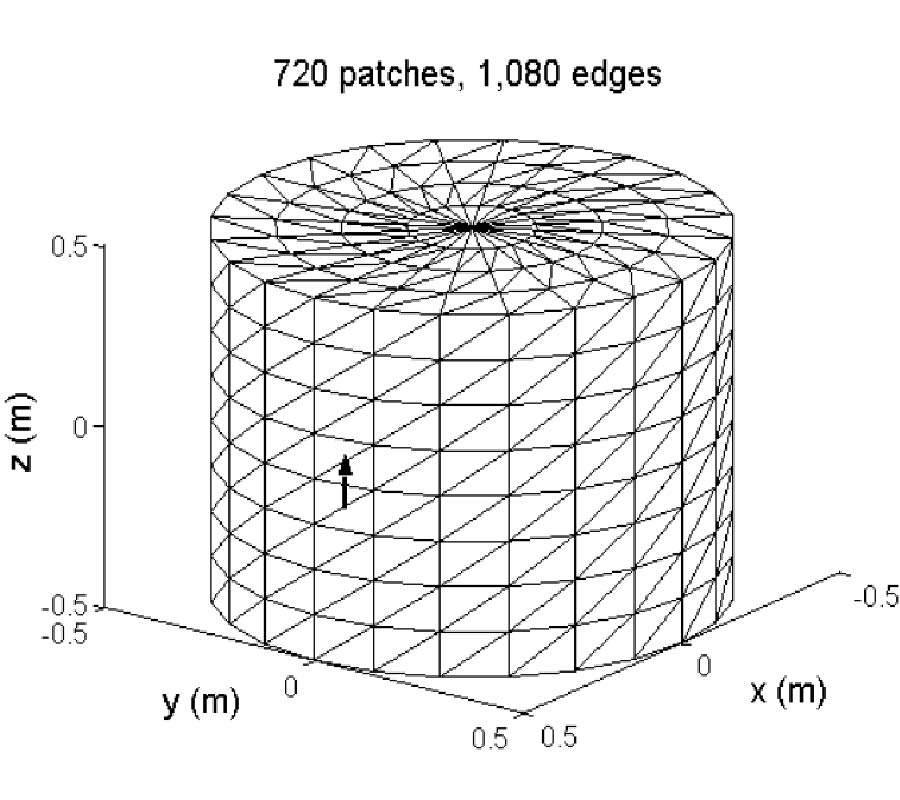Time-Domain EFIE, MFIE, and CFIE Formulations Using Laguerre Polynomials as Temporal Basis Functions for the Analysis of Transient Scattering from Arbitrary Shaped Conducting Structures

In this paper, we present time-domain integral equation (TDIE) formulations for analyzing transient electromagnetic responses from three-dimensional (3-D) arbitrary shaped closed conducting bodies using the time-domain electric field integral equation (TDEFIE), the time-domain magnetic field integral equation (TD-MFIE), and the time-domain combined field integral equation (TD-CFIE). Instead of the conventional marching-on in time (MOT) technique, the solution methods in this paper are based on the Galerkin's method that involves separate spatial and temporal testing procedure. Triangular patch basis functions are used for spatial expansion and testing functions for arbitrarily shaped 3-D structures. The timedomain unknown coefficient is approximated by using an orthonormal basis function set that is derived from the Laguerre functions. These basis functions are also used as temporal testing. Using these Laguerre functions it is possible to evaluate the time derivatives in an analytic fashion. We also propose a second alternative formulation to solve the TDIE. The methods to be described result in very accurate and stable transient responses from conducting objects. Detailed mathematical steps are included and representative numerical results are presented and compared.